Courses

# MCQ : Quadratic Equations - 1

## 10 Questions MCQ Test Mathematics (Maths) Class 10 | MCQ : Quadratic Equations - 1

Description
This mock test of MCQ : Quadratic Equations - 1 for Class 10 helps you for every Class 10 entrance exam. This contains 10 Multiple Choice Questions for Class 10 MCQ : Quadratic Equations - 1 (mcq) to study with solutions a complete question bank. The solved questions answers in this MCQ : Quadratic Equations - 1 quiz give you a good mix of easy questions and tough questions. Class 10 students definitely take this MCQ : Quadratic Equations - 1 exercise for a better result in the exam. You can find other MCQ : Quadratic Equations - 1 extra questions, long questions & short questions for Class 10 on EduRev as well by searching above.
QUESTION: 1

### Which of the following is a quadratic equation?

Solution:

A quadratic equation (from the Latin quadratus for "square") is any equation that can be rearranged in standard form as. where x represents an unknown, and a, b, and c represent known numbers, where a ≠ 0. If a = 0, then the equation is linear, not quadratic, as there is no. term.

Therefore, x3 - x2 = ( x -1)3 is a quadratic equation.

QUESTION: 2

### Which of the following is not a quadratic equation?

Solution: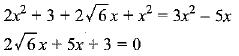QUESTION: 3

### Which of the following equations has 2 as a root?

Solution:
QUESTION: 4

Which of the following are the roots of the quadratic equation, x2 - 9x + 20 = 0 by factorisation?

Solution: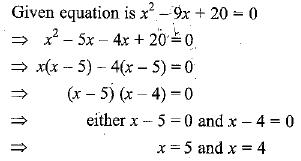∴ x = 4 and 5 are the roots/solution of the given quadratic equation

QUESTION: 5

Value(s) of k for which the quadratic equation 2x2 -kx + k = 0 has equal roots is

Solution:
QUESTION: 6

If p,q and r are rational numbers and p ≠ q ≠ r, then roots of the equation (p2 - q2)x2 - (q2 - r2)x + (r2 - p2) = 0 are

Solution:

Putting x = - 1, we have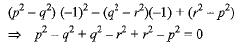∴ x = - 1 is one root.
Only option (d) has one root - 1.

QUESTION: 7

The two consecutive odd positive integers, sum of whose squares is 290 are

Solution:
QUESTION: 8

If α, β are roots of the equation x2 + 5x + 5 = 0, then equation whose roots are α + 1 and β + 1 is

Solution: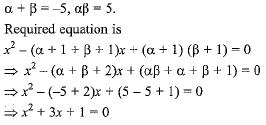QUESTION: 9

The quadratic equation with real co-efficients whose one root is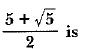Solution:
QUESTION: 10

Which of the following equations has two distinct real roots ?

Solution: